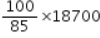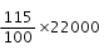# When a plot is sold for Rs. 18,700, the owner loses 15%

When a plot is sold for Rs. 18,700, the owner loses 15%. At what price must that plot be sold in order to gain 15%?

First we find CP.
If SP is Rs. 85 then CP = Rs 100
If SP is Rs. 18,700, then
CP == Rs 22,000
Now, if CP is Rs 100, then SP = Rs. 115
Thus, if CP is Rs. 22,000, then
SP ==Rs 25,300